Next: 4.2.3 Remarks Up: 4.2 Silicon Thin Layers Previous: 4.2.1 Anisotropy in Ultra-Thin Silicon Layers   Contents

## 4.2.2 Analysis and Discussion

The ballistic phonon transmission function and thus the ballistic thermal conductance in the Landauer formalism are determined by the product of the density of states and the group velocity (Eq. 2.42). In Fig. 4.11 we plot the density of states for thin-layers of thickness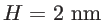with four different surface orientations. Although some differences are observed for the different surface orientations, especially in the low frequency range, the overall values and trends are very similar. The inset of Fig. 4.11 shows the density of states for layers of thickness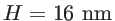. In this case a much smaller variation is observed as expected, since the phonon density of states depends on the number of atoms, and layers of the same thickness contain a similar amount of atoms. At smaller thicknesses the different arrangement of atoms can result in slightly different numbers of atoms for different surfaces, but as the thickness increases the crystal becomes more uniform and any variations are eliminated. In general, of course, the arrangement of atoms, the coupling between them, and the type of interactions they have can also influence their density of states. But as we show in Fig. 4.11, such effects on the density of states are only important at very thin sizes, i.e., and even then, they are small. From this one can conclude that the variation in the thermal conductance and transmission does not originate from the difference in the density of states.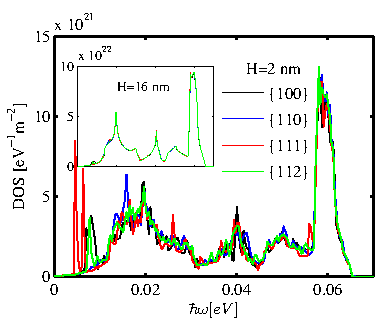In Fig. 4.12 the effective group velocity defined in Eq. 4.1 is plotted. This quantity is orientation-dependent, in contrast to the density of states, and indicates how dispersive the modes are. The velocity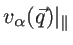is calculated along the transport direction. The density of states times the effective group velocity is proportional to the transmission function. Therefore, the differences in the transmission functions should be seen in the effective group velocities of the channels, since the density of states is the same for all channels of the same thickness. Figure 4.12 shows the effective group velocities of the channels considered. Figures 4.12-a and 4.12-c show the effective group velocities of thin-layers with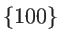and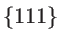surfaces along the two different orientations with the lowest and highest thermal conductance for each surface orientation. The two different cases for each surface are almost identical, as in the case of the transmission functions in Fig. 4.8-a and 4.8-c. Figures 4.12-b and 4.12-d show the effective group velocities for channels with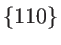andsurface orientations, respectively. A variation is observed for the different channels, which causes the difference in the transmission functions shown earlier in Fig. 4.8-b and 4.8-d.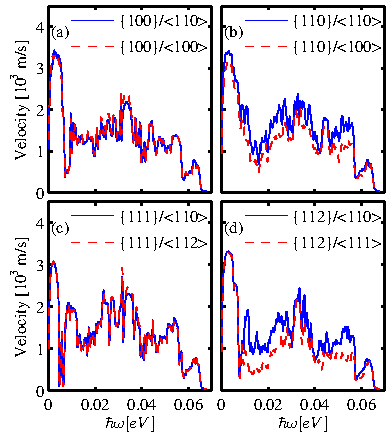The anisotropy of the effective group velocity originates from the phonon bandstructure. In Fig. 4.13 the contour plots of the phonon bandstructure at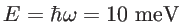is shown for the eight channels considered in Fig. 4.8 and Fig. 4.12 for layer thickness. This is an energy value at which the most significant differences for the channels withandsurfaces appear. It turns out that what is presented for this energy is a good indicator of the anisotropic behavior of the entire energy spectrum, most of which contributes to thermal conductance at room temperature. The lines represent the different modes at that energy, whereas the colormap indicates the cumulative ballistic thermal conductance at room temperature in the transport orientation of the specific channel of interest, as indicated by the arrow in each case. Elongation of contour lines along a specific direction provides high phonon group velocities in the perpendicular direction, and consequently high thermal conductance. This is very similar to the low effective mass and high velocities of carriers in an ellipsoidal band along the direction of the short axis.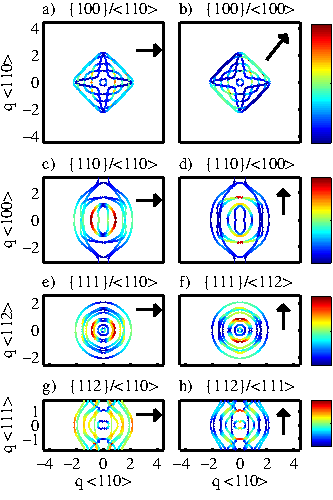Figures 4.13-a and 4.13-b show the energy contours for thesurface in the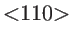and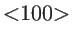transport orientations, respectively (indicated by the arrow). Despite the square like shape of the contour, which indicates that there is different symmetry in the two orientations of interest, the contours are elongated similarly in both directions, which results in a similar thermal conductance for both channels. This is the case for almost the entire energy spectrum (although at higher energies there are many more modes and more complex contour shapes). In the case of thesurface in Fig. 4.13-e and 4.13-f, a highly symmetric contour provides very similar transmission functions and thermal conductances along theand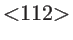directions.

The largest differences in the thermal conductance are observed for thin layers withandsurfaces in Fig. 4.13-c, 4.13-d and Fig. 4.13-g, 4.13-h, respectively. In both cases, the contours at energy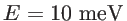are clearly elongated along the vertical axis. This results in a larger phonon group velocity along the horizontal axis, and finally a higher thermal conductance, as also indicated by the colormap. This is especially evident for thesurface, where the contour of thechannel in Fig. 4.13-g is colored much closer to red (higher conductance value) than the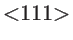channel in Fig. 4.13-h, indicating much larger phonon group velocities. This causes the thermal transmission and conductance of the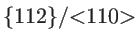channel to be higher than that of the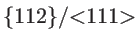channel shown in Fig. 4.8-d and Fig. 4.9-d.

Figure 4.13 explains the origin of anisotropy in the ballistic thermal conductance of thin-layers with 2nm thickness. However, such effects also hold for all the thicknesses examined, i.e. up to. The anisotropic behavior depends weakly on the layer thickness. The ballistic conductance increases linearly as the layer thickness increases due to the increased number of atoms which results in a larger number of phonon modes, but the anisotropy does not change significantly. This is illustrated in Fig. 4.14-a which shows the ballistic thermal conductance for each of the four surface orientations examined, normalized by the thickness of the layer. For each surface only the direction showing the maximum conductance is considered. The results show that in all cases the normalized conductance is constant, even down to a thickness of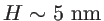. Below, variations of the order ofare observed for all channels. From this, it follows that other than the reduction in the size of the phonon spectrum with thickness scaling, no significant changes in the shape of the phonon structure are observed, at least not significant enough to introduce changes in the thermal conductance. This is also supported by Fig. 4.14-b, which depicts the ratio of the maximum to the minimum thermal conductance that can be achieved for each surface. Similarly to Fig. 4.14-a, the anisotropy does not change with layer thickness even down to. Again, below, differences of the order of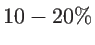can be observed.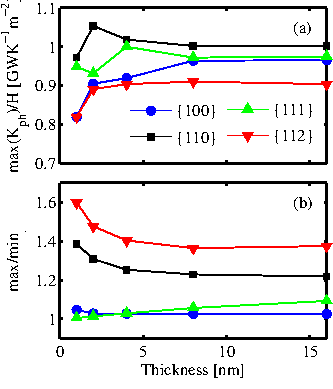This anisotropy observed is not only a function of thickness, but also of temperature. Figure 4.15 shows the ratio of the maximum to the minimum ballistic thermal conductance for the four surface orientations of interest, again by choosing the appropriate transport orientations. Figure 4.15-a and 4.15-b show results forand, respectively. The maximum anisotropy (up to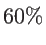) is observed for thesurface, followed by thesurface (up to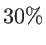), whereas the thermal conductance for theandsurfaces are more or less isotropic (the ratio stays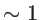). This holds for most of the temperature range examined, even down to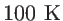. Below, the ratio approaches unity in all cases, because at this temperature the main contribution to thermal conductance comes from the acoustic branches at low energy, which are more isotropic. This is clearly observed in the low energy range of the transmissions in Fig. 4.8, in which the thermal conductivity is isotropic.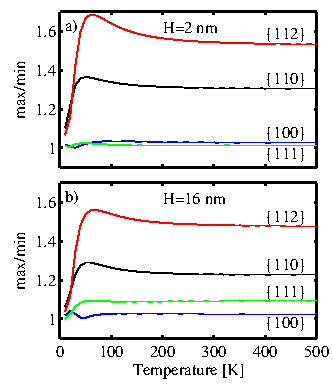Next: 4.2.3 Remarks Up: 4.2 Silicon Thin Layers Previous: 4.2.1 Anisotropy in Ultra-Thin Silicon Layers   Contents
H. Karamitaheri: Thermal and Thermoelectric Properties of Nanostructures